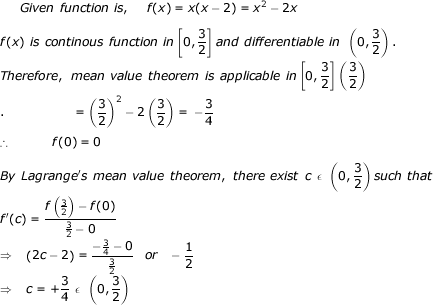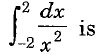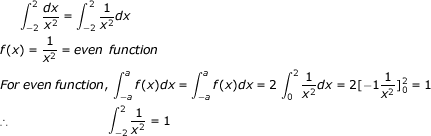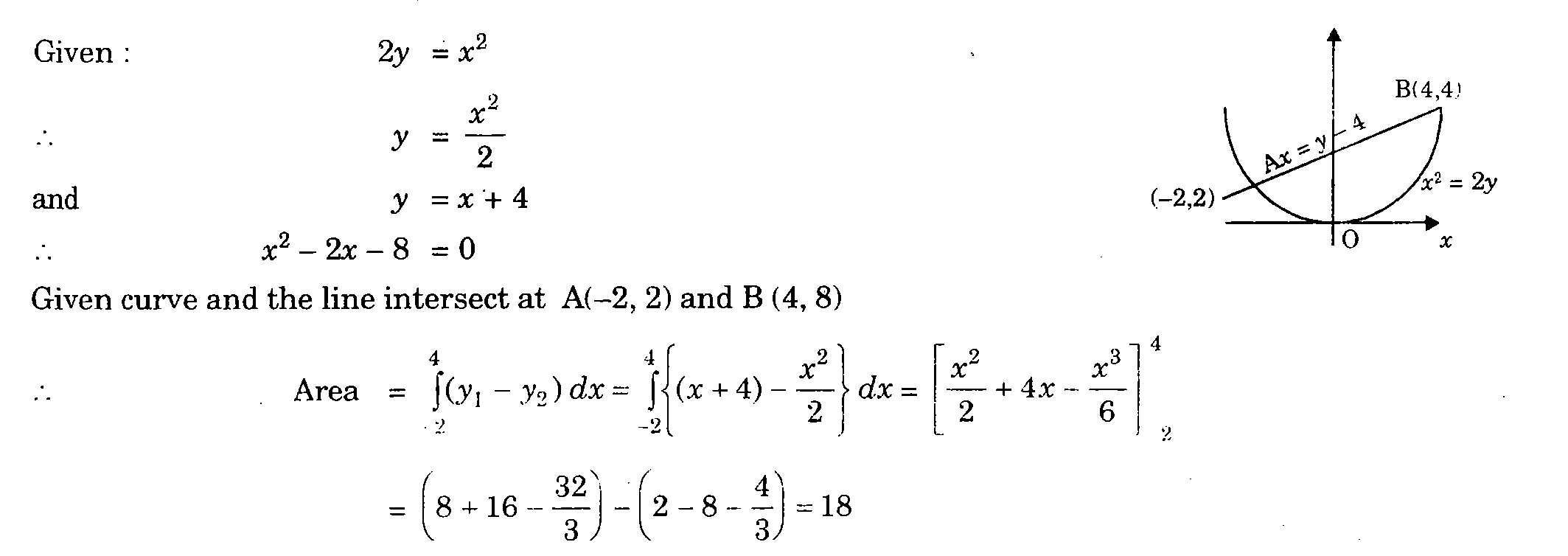# Calculus - Calculus MCQ

21:

Value of 'c' of the mean value theorem for the function f(x) = x(x - 2), when a = 0, b = 3/2, is

 A. 3/4 B. 1/2 C. 3/2 D. 1/4 Answer Report Discuss Option: A Explanation :Click on Discuss to view users comments. Write your comments here:
22:

If f(x , y) = x2 + y2, then ∇2 f is

 A. 4 B. 2 C. 0 D. 4(x + y)2 Answer Report Discuss Option: A Explanation :Click on Discuss to view users comments. Write your comments here:
23:

The value of integralA. 0 B. 0.25 C. 1 D. ∞ Answer Report Discuss Option: C Explanation :Click on Discuss to view users comments. T. Singh said: (7:06am on Thursday 9th November 2017) Option D should be correct as integral of 1/x2 is -1*(1/x) . Substitution of limits 2 and 0 will result as -1/2 infinity. So option D should be correct option. Write your comments here:
24:

Angle between two unit-magnitude coplanar vectors P(0.866, 0.500, 0) and Q(0.259, 0.966, 0) will be

 A. 00 B. 300 C. 450 D. 600 Answer Report Discuss Option: C Explanation :Click on Discuss to view users comments. Write your comments here:
25:

Area bounded by the parabola 2y = x2 and the line x = y - 4 is equal to

 A. 6 B. 18 C. ∞ D. none of these Answer Report Discuss Option: B Explanation :Click on Discuss to view users comments. Write your comments here: